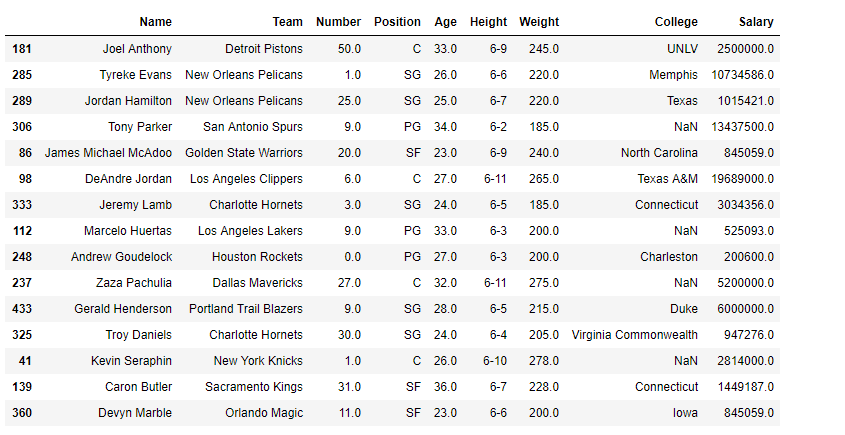Open In App

# Python | Pandas dataframe.sort_index()

Python is a great language for doing data analysis, primarily because of the fantastic ecosystem of data-centric python packages. Pandas is one of those packages and makes importing and analyzing data much easier.
Pandas dataframe.sort_index() function sorts objects by labels along the given axis.
Basically the sorting algorithm is applied on the axis labels rather than the actual data in the dataframe and based on that the data is rearranged. We have the freedom to choose what sorting algorithm we would like to apply. There are three possible sorting algorithms that we can use ‘quicksort’, ‘mergesort’ and ‘heapsort’.

Syntax: DataFrame.sort_index(axis=0, level=None, ascending=True, inplace=False, kind=’quicksort’, na_position=’last’, sort_remaining=True, by=None)
Parameters :
axis : index, columns to direct sorting
level : if not None, sort on values in specified index level(s)
ascending : Sort ascending vs. descending
inplace : if True, perform operation in-place
kind : {‘quicksort’, ‘mergesort’, ‘heapsort’}, default ‘quicksort’. Choice of sorting algorithm. See also ndarray.np.sort for more information. mergesort is the only stable algorithm. For DataFrames, this option is only applied when sorting on a single column or label.
na_position : [{‘first’, ‘last’}, default ‘last’] First puts NaNs at the beginning, last puts NaNs at the end. Not implemented for MultiIndex.
sort_remaining : If true and sorting by level and index is multilevel, sort by other levels too (in order) after sorting by specified level
Return : sorted_obj : DataFrame

Example #1: Use sort_index() function to sort the dataframe based on the index labels.

## Python3

 `# importing pandas as pd``import` `pandas as pd` `# Creating the dataframe``df ``=` `pd.read_csv(``"nba.csv"``)` `# Print the dataframe``df`As we can see in the output, the index labels are already sorted i.e. (0, 1, 2, ….). So we are going to extract a random sample out of it and then sort it for the demonstration purpose.
Lets extract a random sample of 15 elements from the dataframe using dataframe.sample() function.

## Python3

 `# extract the sample dataframe from "df"``# and store it in "sample_df"``sample_df ``=` `df.sample(``15``)` `# Print the sample data frame``sample_df`Note : Every time we execute dataframe.sample() function, it will give different output. Let’s use the dataframe.sort_index() function to sort the dataframe based on the index labels

## Python3

 `# sort by index labels``sample_df.sort_index(axis ``=` `0``)`

Output :As we can see in the output, the index labels are sorted.

Example #2: Use sort_index() function to sort the dataframe based on the column labels.

## Python3

 `# importing pandas as pd``import` `pandas as pd` `# Creating the dataframe``df ``=` `pd.read_csv(``"nba.csv"``)` `# sorting based on column labels``df.sort_index(axis ``=` `1``)`

Output :